Functional RL with Keras and Tensorflow Eager
berkeley_ai

In this blog post, we explore a functional paradigm for implementing reinforcement learning (RL) algorithms. The paradigm will be that developers write the numerics of their algorithm as independent, pure functions, and then use a library to compile them into policies that can be trained at scale. We share how these ideas were implemented in RLlib’s policy builder API, eliminating thousands of lines of “glue” code and bringing support for Keras and TensorFlow 2.0.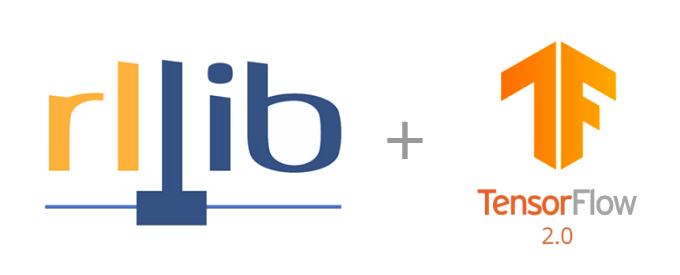### Why Functional Programming?

One of the key ideas behind functional programming is that programs can be composed largely of pure functions, i.e., functions whose outputs are entirely determined by their inputs. Here less is more: by imposing restrictions on what functions can do, we gain the ability to more easily reason about and manipulate their execution.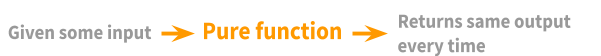In TensorFlow, such functions of tensors can be executed either symbolically with placeholder inputs or eagerly with real tensor values. Since such functions have no side-effects, they have the same effect on inputs whether they are called once symbolically or many times eagerly.

### Functional Reinforcement Learning

Consider the following loss function over agent rollout data, with current state $s$, actions $a$, returns $r$, and policy $\pi$:

If you’re not familiar with RL, all this function is saying is that we should try to improve the probability of good actions (i.e., actions that increase the future returns). Such a loss is at the core of policy gradient algorithms. As we will see, defining the loss is almost all you need to start training a RL policy in RLlib.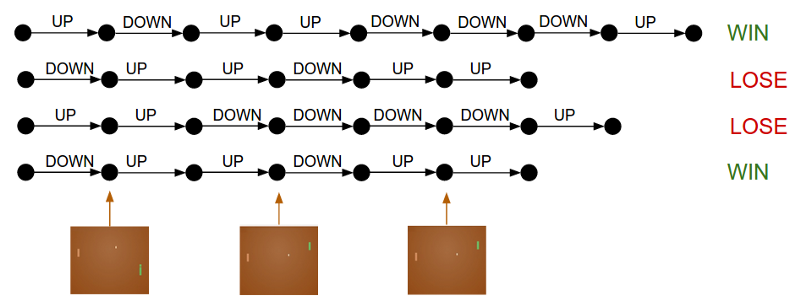Given a set of rollouts, the policy gradient loss seeks to improve the probability of good actions (i.e., those that lead to a win in this Pong example above).

A straightforward translation into Python is as follows. Here, the loss function takes $(\pi, s, a, r)$, computes $\pi(s, a)$ as a discrete action distribution, and returns the log probability of the actions multiplied by the returns:

def loss(model, s: Tensor, a:  Tensor, r: Tensor) -> Tensor:
logits = model.forward(s)
action_dist = Categorical(logits)
return -tf.reduce_mean(action_dist.logp(a) * r)


There are multiple benefits to this functional definition. First, notice that loss reads quite naturally — there are no placeholders, control loops, access of external variables, or class members as commonly seen in RL implementations. Second, since it doesn’t mutate external state, it is compatible with both TF graph and eager mode execution.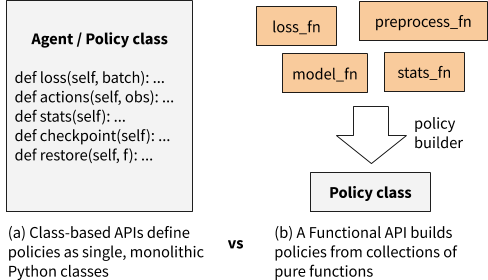In contrast to a class-based API, in which class methods can access arbitrary parts of the class state, a functional API builds policies from loosely coupled pure functions.

In this blog we explore defining RL algorithms as collections of such pure functions. The paradigm will be that developers write the numerics of their algorithm as independent, pure functions, and then use a RLlib helper function to compile them into policies that can be trained at scale. This proposal is implemented concretely in the RLlib library.

### Functional RL with RLlib

RLlib is an open-source library for reinforcement learning that offers both high scalability and a unified API for a variety of applications. It offers a wide range of scalable RL algorithms.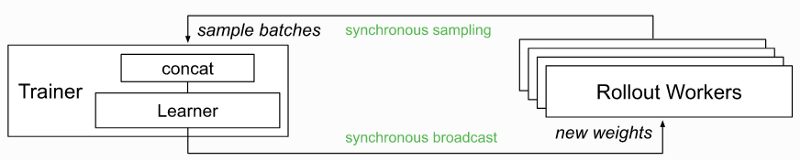Example of how RLlib scales algorithms, in this case with distributed synchronous sampling.

Given the increasing popularity of PyTorch (i.e., imperative execution) and the imminent release of TensorFlow 2.0, we saw the opportunity to improve RLlib’s developer experience with a functional rewrite of RLlib’s algorithms. The major goals were to:

Improve the RL debugging experience

• Allow eager execution to be used for any algorithm with just an — eager flag, enabling easy print() debugging.

Simplify new algorithm development

• Make algorithms easier to customize and understand by replacing monolithic “Agent” classes with policies built from collections of pure functions (e.g., primitives provided by TRFL).
• Remove the need to manually declare tensor placeholders for TF.
• Unify the way TF and PyTorch policies are defined.

### Policy Builder API

The RLlib policy builder API for functional RL (stable in RLlib 0.7.4) involves just two key functions:

At a high level, these builders take a number of function objects as input, including a loss_fn similar to what you saw earlier, a model_fn to return a neural network model given the algorithm config, and an action_fn to generate action samples given model outputs. The actual API takes quite a few more arguments, but these are the main ones. The builder compiles these functions into a policy that can be queried for actions and improved over time given experiences: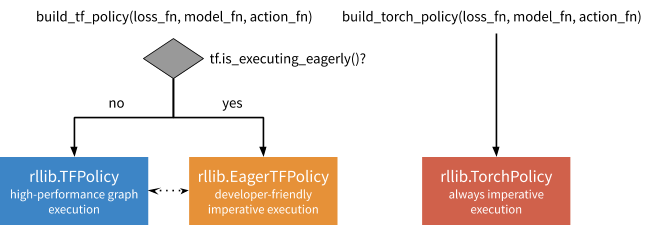These policies can be leveraged for single-agent, vector, and multi-agent training in RLlib, which calls on them to determine how to interact with environments: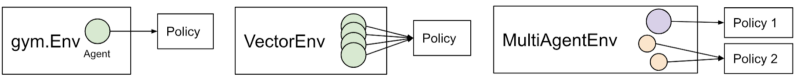We’ve found the policy builder pattern general enough to port almost all of RLlib’s reference algorithms, including A2C, APPO, DDPG, DQN, PG, PPO, SAC, and IMPALA in TensorFlow, and PG / A2C in PyTorch. While code readability is somewhat subjective, users have reported that the builder pattern makes it much easier to customize algorithms, especially in environments such as Jupyter notebooks. In addition, these refactorings have reduced the size of the algorithms by up to hundreds of lines of code each.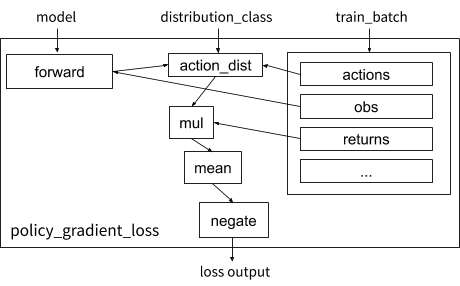Visualization of the vanilla policy gradient loss function in RLlib.

Let’s take a look at how the earlier loss example can be implemented concretely using the builder pattern. We define policy_gradient_loss, which requires a couple of tweaks for generality: (1) RLlib supplies the proper distribution_class so the algorithm can work with any type of action space (e.g., continuous or categorical), and (2) the experience data is held in a train_batch dict that contains state, action, etc. tensors:

def policy_gradient_loss(
policy, model, distribution_cls, train_batch):
logits, _ = model.from_batch(train_batch)
action_dist = distribution_cls(logits, model)
return -tf.reduce_mean(
action_dist.logp(train_batch[“actions”]) *
train_batch[“returns”])


To add the “returns” array to the batch, we need to define a postprocessing function that calculates it as the temporally discounted reward over the trajectory:

We set $\gamma = 0.99$ when computing $R(T)$ below in code:

from ray.rllib.evaluation.postprocessing import discount

# Run for each trajectory collected from the environment
def calculate_returns(policy,
batch,
other_agent_batches=None,
episode=None):
batch[“returns”] = discount(batch[“rewards”], 0.99)
return batch


Given these functions, we can then build the RLlib policy and trainer (which coordinates the overall training workflow). The model and action distribution are automatically supplied by RLlib if not specified:

MyTFPolicy = build_tf_policy(
name="MyTFPolicy",
postprocess_fn=calculate_returns)

MyTrainer = build_trainer(
name="MyCustomTrainer", default_policy=MyTFPolicy)


Now we can run this at the desired scale using Tune, in this example showing a configuration using 128 CPUs and 1 GPU in a cluster:

tune.run(MyTrainer,
config={“env”: “CartPole-v0”,
“num_workers”: 128,
“num_gpus”: 1})


While this example (runnable code) is only a basic algorithm, it demonstrates how a functional API can be concise, readable, and highly scalable. When compared against the previous way to define policies in RLlib using TF placeholders, the functional API uses ~3x fewer lines of code (23 vs 81 lines), and also works in eager: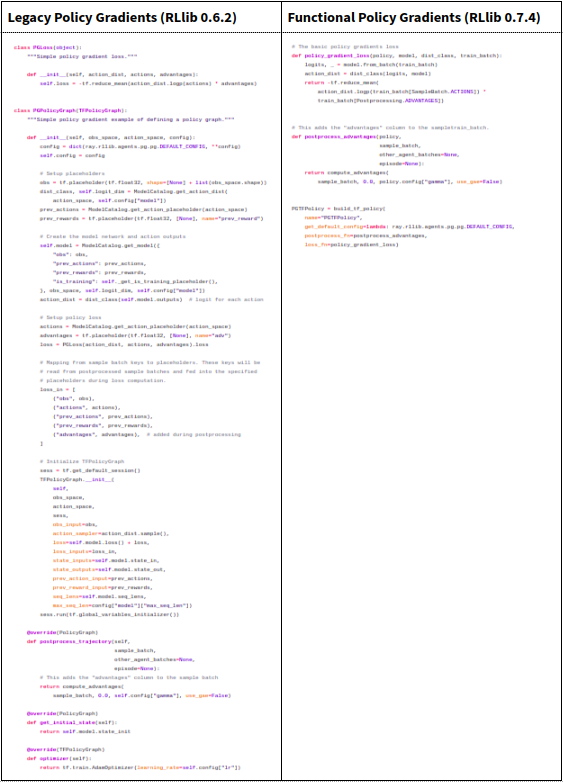Comparing the legacy class-based API with the new functional policy builder API Both policies implement the same behaviour, but the functional definition is much shorter.

### How the Policy Builder works

Under the hood, build_tf_policy takes the supplied building blocks (model_fn, action_fn, loss_fn, etc.) and compiles them into either a DynamicTFPolicy or EagerTFPolicy, depending on if TF eager execution is enabled. The former implements graph-mode execution (auto-defining placeholders dynamically), the latter eager execution.

The main difference between DynamicTFPolicy and EagerTFPolicy is how many times they call the functions passed in. In either case, a model_fn is invoked once to create a Model class. However, functions that involve tensor operations are either called once in graph mode to build a symbolic computation graph, or multiple times in eager mode on actual tensors. In the following figures we show how these operations work together in blue and orange: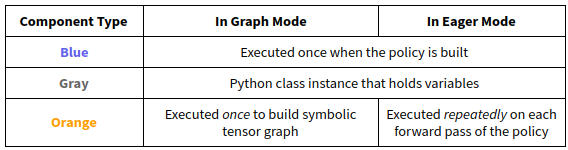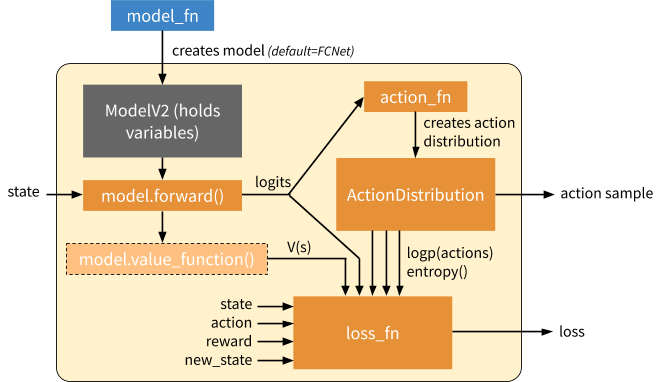Overview of a generated EagerTFPolicy. The policy passes the environment state through model.forward(), which emits output logits. The model output parameterizes a probability distribution over actions (“ActionDistribution”), which can be used when sampling actions or training. The loss function operates over batches of experiences. The model can provide additional methods such as a value function (light orange) or other methods for computing Q values, etc. (not shown) as needed by the loss function.

This policy object is all RLlib needs to launch and scale RL training. Intuitively, this is because it encapsulates how to compute actions and improve the policy. External state such as that of the environment and RNN hidden state is managed externally by RLlib, and does not need to be part of the policy definition. The policy object is used in one of two ways depending on whether we are computing rollouts or trying to improve the policy given a batch of rollout data: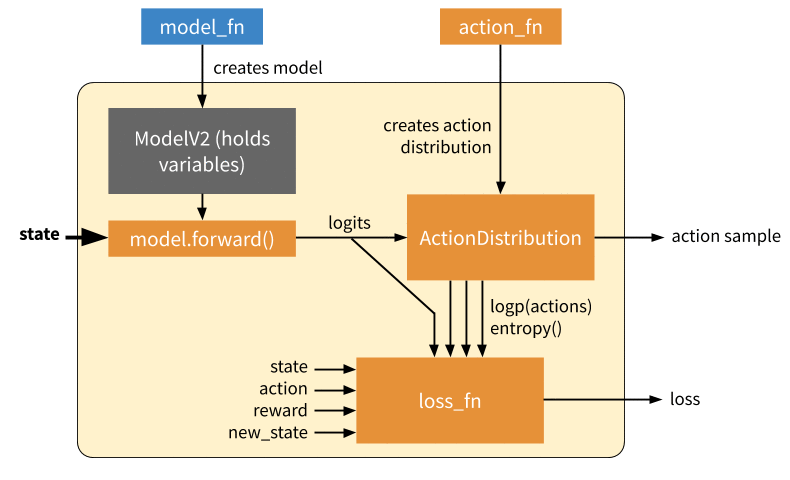Inference: Forward pass to compute a single action. This only involves querying the model, generating an action distribution, and sampling an action from that distribution. In eager mode, this involves calling action_fn DQN example of an action sampler, which creates an action distribution / action sampler as relevant that is then sampled from.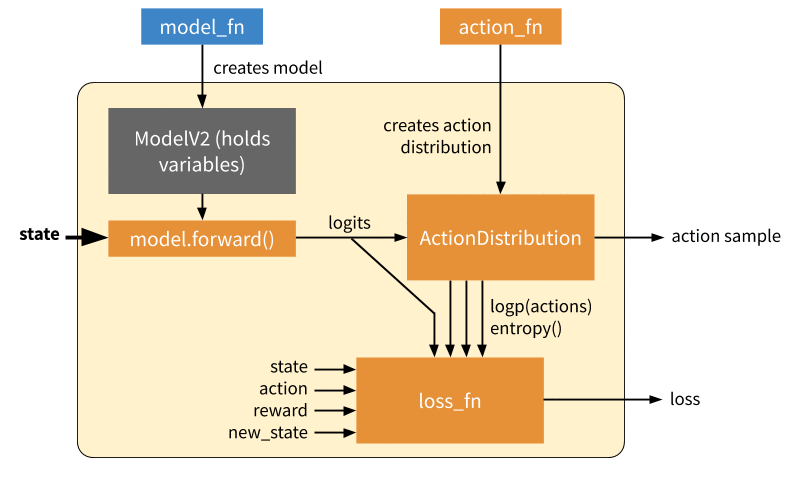Training: Forward and backward pass to learn on a batch of experiences. In this mode, we call the loss function to generate a scalar output which can be used to optimize the model variables via SGD. In eager mode, both action_fn and loss_fn are called to generate the action distribution and policy loss respectively. Note that here we don’t show differentiation through action_fn, but this does happen in algorithms such as DQN.

### Loose Ends: State Management

RL training inherently involves a lot of state. If algorithms are defined using pure functions, where is the state held? In most cases it can be managed automatically by the framework. There are three types of state that need to be managed in RLlib:

1. Environment state: this includes the current state of the environment and any recurrent state passed between policy steps. RLlib manages this internally in its rollout worker implementation.
2. Model state: these are the policy parameters we are trying to learn via an RL loss. These variables must be accessible and optimized in the same way for both graph and eager mode. Fortunately, Keras models can be used in either mode. RLlib provides a customizable model class (TFModelV2) based on the object-oriented Keras style to hold policy parameters.
3. Training workflow state: state for managing training, e.g., the annealing schedule for various hyperparameters, steps since last update, and so on. RLlib lets algorithm authors add mixin classes to policies that can hold any such extra variables.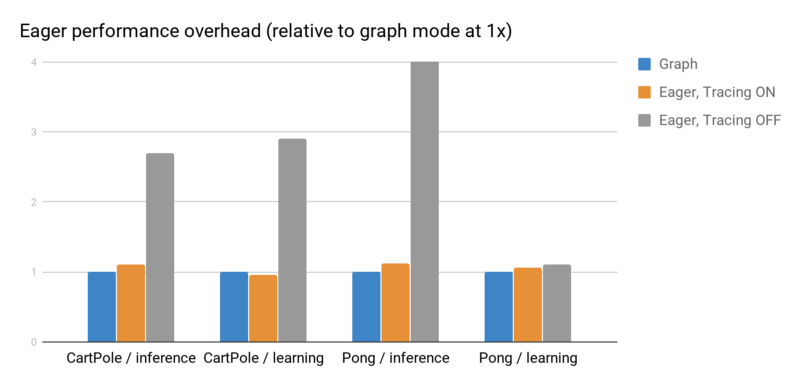Eager inference and gradient overheads measured using rllib train --run=PG --env=<env> [ --eager [ --trace]] on a laptop processor. With tracing off, eager imposes a significant overhead for small batch operations. However it is often as fast or faster than graph mode when tracing is enabled.
To recap, in this blog post we propose using ideas from functional programming to simplify the development of RL algorithms. We implement and validate these ideas in RLlib. Beyond making it easy to support new features such as eager execution, we also find the functional paradigm leads to substantially more concise and understandable code. Try it out yourself with pip install ray[rllib] or by checking out the docs and source code.